# Selina Solutions Concise Maths Class 10 Chapter 20 Cylinder, Cone and Sphere (Surface Area and Volume) Exercise 20(E)

When solids are combined together by surmounting or mounting we get a new solid. In this exercise, students will learn to find the surface areas and volumes of these combinations of solids. To attain better understanding over concepts and to develop strong problem-solving skills, the Selina Solutions for Class 10 Maths is the tool for it. The solution PDF of the Concise Selina Solutions for Class 10 Maths Chapter 20 Cylinder, Cone and Sphere (Surface Area and Volume) Exercise 20(E) is provided in the link below.

## Selina Solutions Concise Maths Class 10 Chapter 20 Cylinder, Cone and Sphere (Surface Area and Volume) Exercise 20(E) Download PDF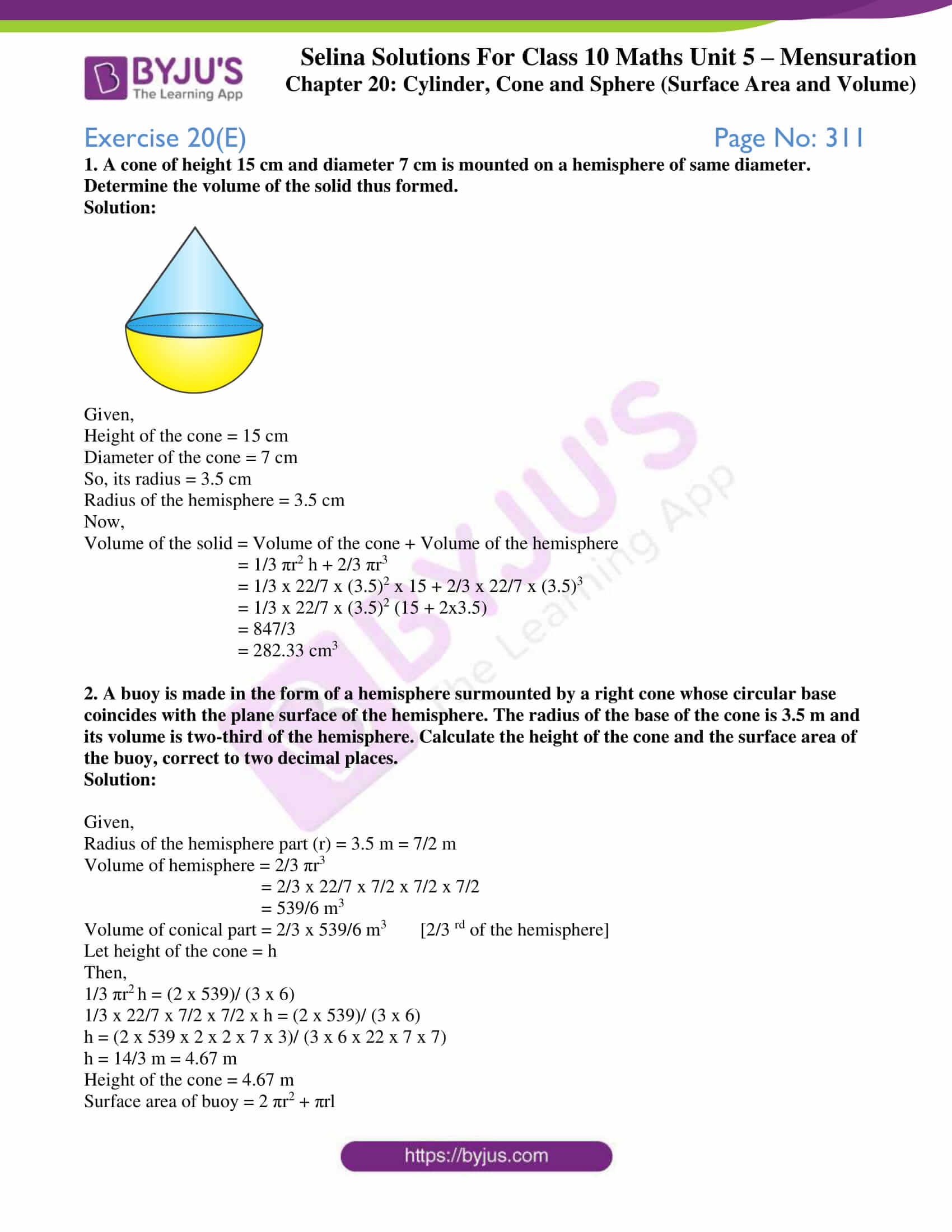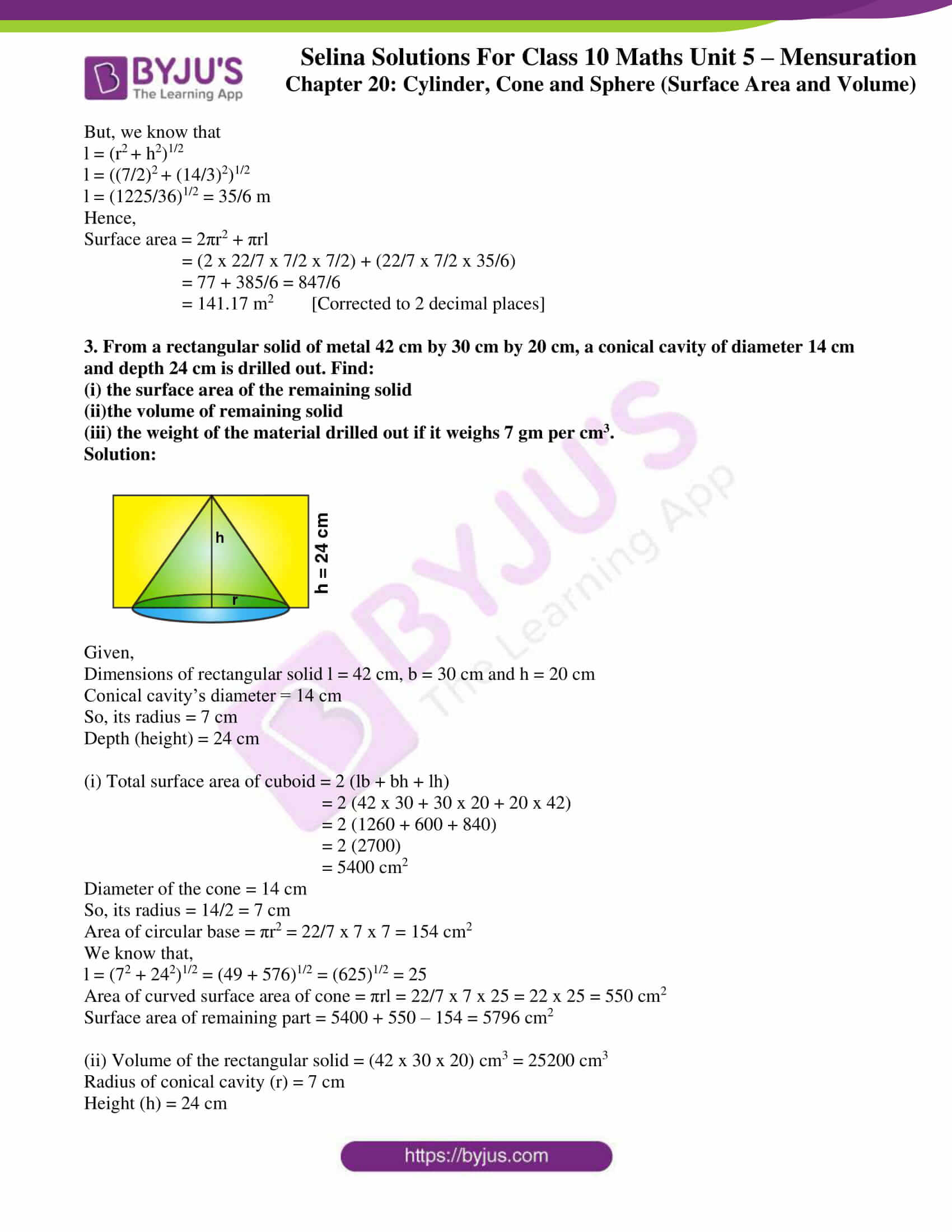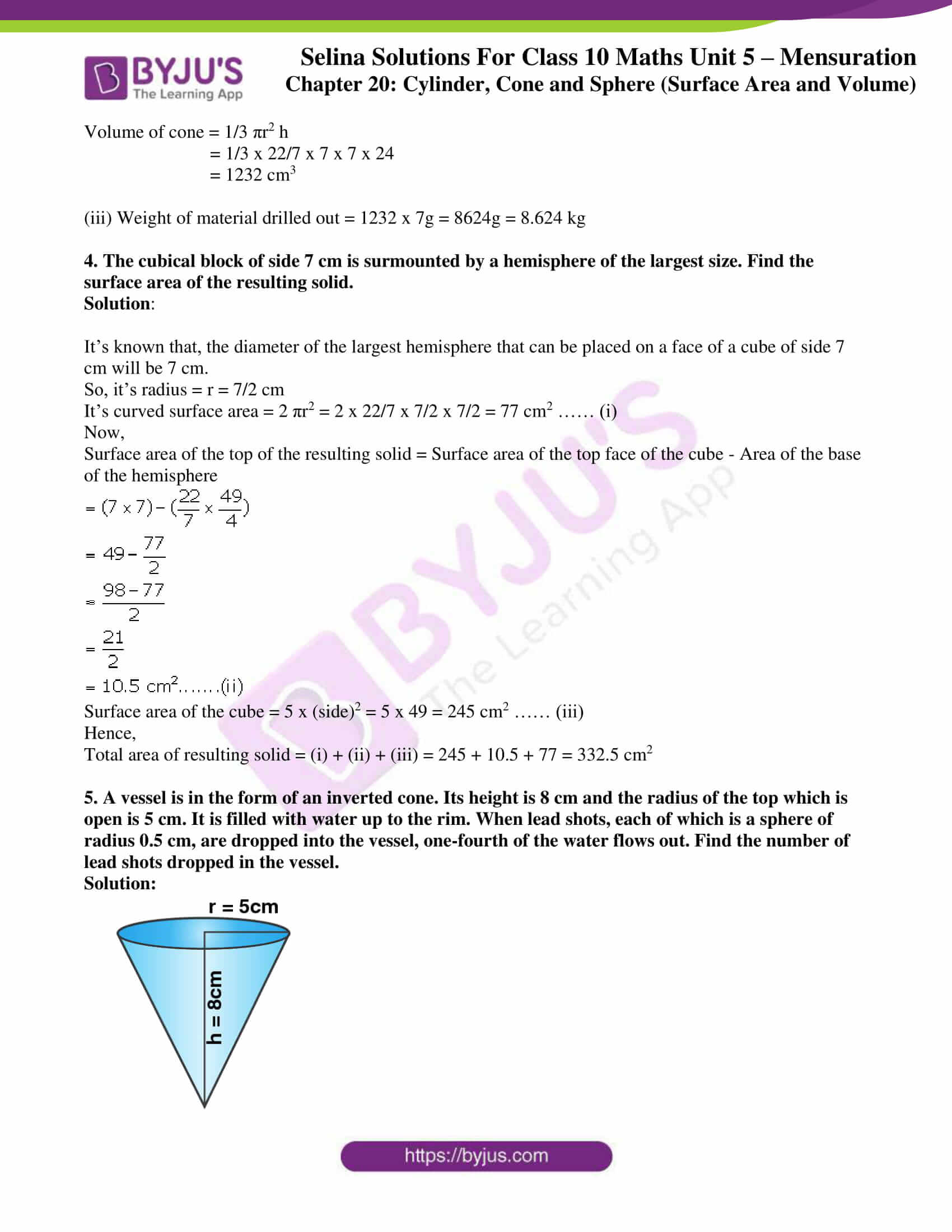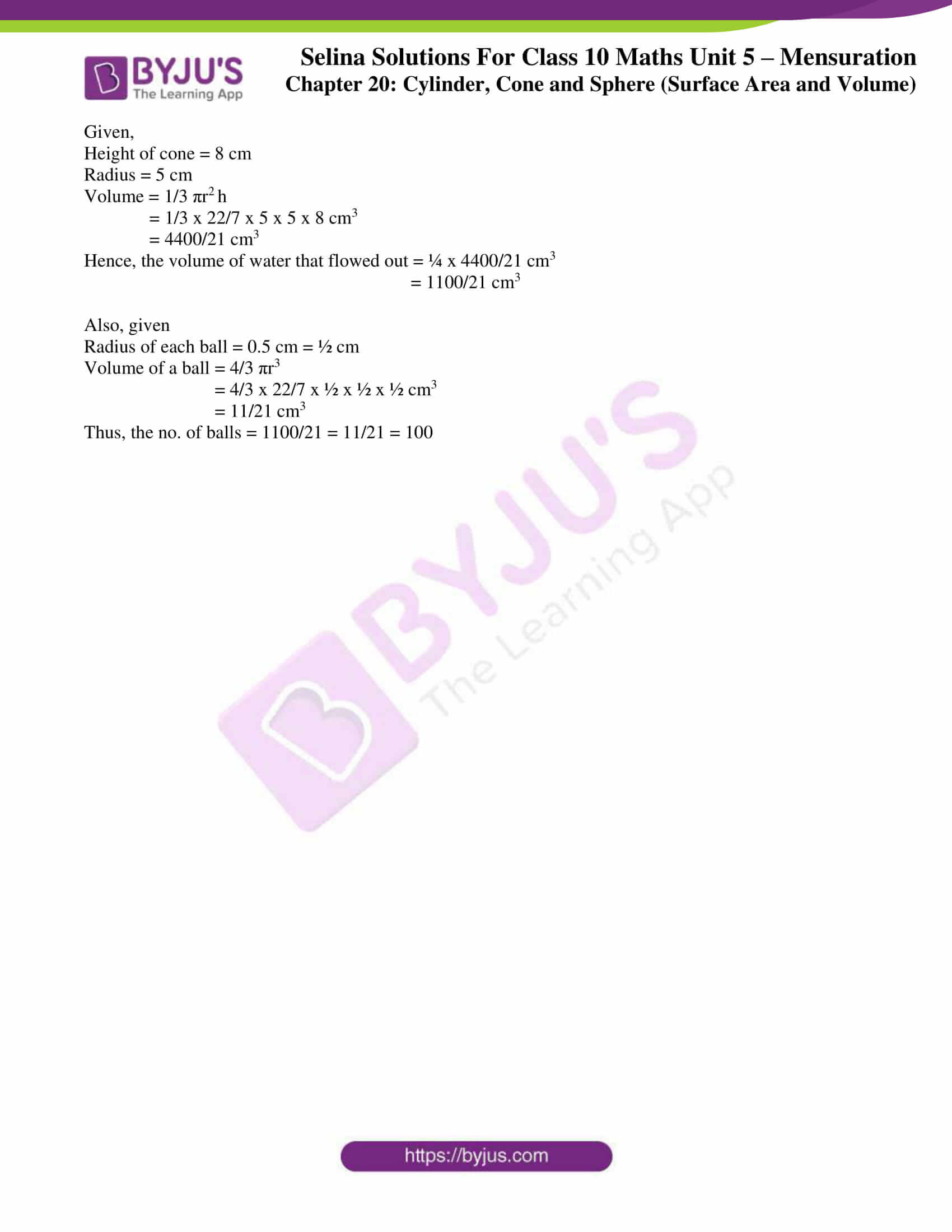### Access Selina Solutions Concise Maths Class 10 Chapter 20 Cylinder, Cone and Sphere (Surface Area and Volume) Exercise 20(E)

1. A cone of height 15 cm and diameter 7 cm is mounted on a hemisphere of same diameter. Determine the volume of the solid thus formed.

Solution: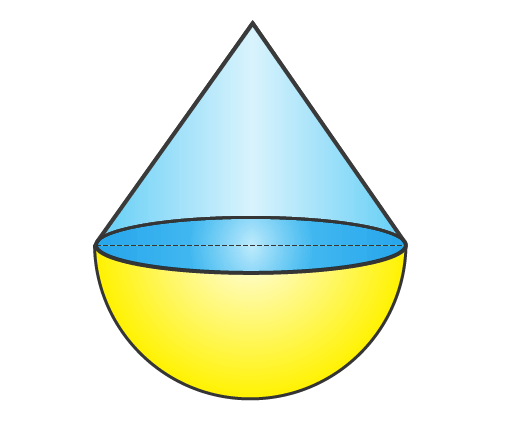Given,

Height of the cone = 15 cm

Diameter of the cone = 7 cm

So, its radius = 3.5 cm

Radius of the hemisphere = 3.5 cm

Now,

Volume of the solid = Volume of the cone + Volume of the hemisphere

= 1/3 πr2 h + 2/3 πr3

= 1/3 x 22/7 x (3.5)2 x 15 + 2/3 x 22/7 x (3.5)3

= 1/3 x 22/7 x (3.5)2 (15 + 2×3.5)

= 847/3

= 282.33 cm3

2. A buoy is made in the form of a hemisphere surmounted by a right cone whose circular base coincides with the plane surface of the hemisphere. The radius of the base of the cone is 3.5 m and its volume is two-third of the hemisphere. Calculate the height of the cone and the surface area of the buoy, correct to two decimal places.

Solution:

Given,

Radius of the hemisphere part (r) = 3.5 m = 7/2 m

Volume of hemisphere = 2/3 πr3

= 2/3 x 22/7 x 7/2 x 7/2 x 7/2

= 539/6 m3

Volume of conical part = 2/3 x 539/6 m3 [2/3 rd of the hemisphere]

Let height of the cone = h

Then,

1/3 πr2 h = (2 x 539)/ (3 x 6)

1/3 x 22/7 x 7/2 x 7/2 x h = (2 x 539)/ (3 x 6)

h = (2 x 539 x 2 x 2 x 7 x 3)/ (3 x 6 x 22 x 7 x 7)

h = 14/3 m = 4.67 m

Height of the cone = 4.67 m

Surface area of buoy = 2 πr2 + πrl

But, we know that

l = (r2 + h2)1/2

l = ((7/2)2 + (14/3)2)1/2

l = (1225/36)1/2 = 35/6 m

Hence,

Surface area = 2πr2 + πrl

= (2 x 22/7 x 7/2 x 7/2) + (22/7 x 7/2 x 35/6)

= 77 + 385/6 = 847/6

= 141.17 m2 [Corrected to 2 decimal places]

3. From a rectangular solid of metal 42 cm by 30 cm by 20 cm, a conical cavity of diameter 14 cm and depth 24 cm is drilled out. Find:

(i) the surface area of the remaining solid

(ii)the volume of remaining solid

(iii) the weight of the material drilled out if it weighs 7 gm per cm3.

Solution: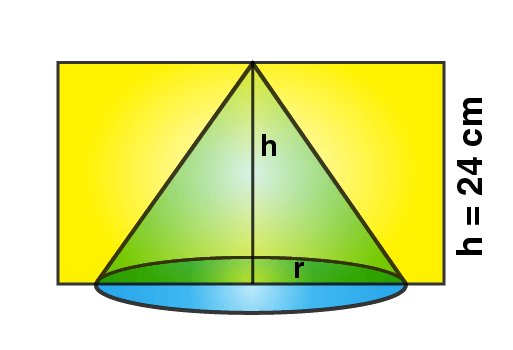Given,

Dimensions of rectangular solid l = 42 cm, b = 30 cm and h = 20 cm

Conical cavity’s diameter = 14 cm

So, its radius = 7 cm

Depth (height) = 24 cm

(i) Total surface area of cuboid = 2 (lb + bh + lh)

= 2 (42 x 30 + 30 x 20 + 20 x 42)

= 2 (1260 + 600 + 840)

= 2 (2700)

= 5400 cm2

Diameter of the cone = 14 cm

So, its radius = 14/2 = 7 cm

Area of circular base = πr2 = 22/7 x 7 x 7 = 154 cm2

We know that,

l = (72 + 242)1/2 = (49 + 576)1/2 = (625)1/2 = 25

Area of curved surface area of cone = πrl = 22/7 x 7 x 25 = 22 x 25 = 550 cm2

Surface area of remaining part = 5400 + 550 – 154 = 5796 cm2

(ii) Volume of the rectangular solid = (42 x 30 x 20) cm3 = 25200 cm3

Radius of conical cavity (r) = 7 cm

Height (h) = 24 cm

Volume of cone = 1/3 πr2 h

= 1/3 x 22/7 x 7 x 7 x 24

= 1232 cm3

(iii) Weight of material drilled out = 1232 x 7g = 8624g = 8.624 kg

4. The cubical block of side 7 cm is surmounted by a hemisphere of the largest size. Find the surface area of the resulting solid.

Solution:

It’s known that, the diameter of the largest hemisphere that can be placed on a face of a cube of side 7 cm will be 7 cm.

So, it’s radius = r = 7/2 cm

It’s curved surface area = 2 πr2 = 2 x 22/7 x 7/2 x 7/2 = 77 cm2 …… (i)

Now,

Surface area of the top of the resulting solid = Surface area of the top face of the cube – Area of the base of the hemisphere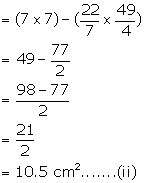Surface area of the cube = 5 x (side)2 = 5 x 49 = 245 cm2 …… (iii)

Hence,

Total area of resulting solid = (i) + (ii) + (iii) = 245 + 10.5 + 77 = 332.5 cm2

5. A vessel is in the form of an inverted cone. Its height is 8 cm and the radius of the top which is open is 5 cm. It is filled with water up to the rim. When lead shots, each of which is a sphere of radius 0.5 cm, are dropped into the vessel, one-fourth of the water flows out. Find the number of lead shots dropped in the vessel.

Solution: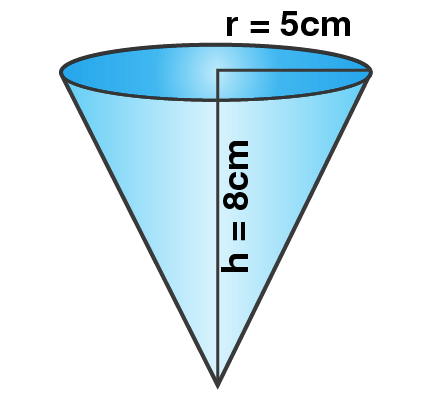Given,

Height of cone = 8 cm

Volume = 1/3 πr2 h

= 1/3 x 22/7 x 5 x 5 x 8 cm3

= 4400/21 cm3

Hence, the volume of water that flowed out = ¼ x 4400/21 cm3

= 1100/21 cm3

Also, given

Radius of each ball = 0.5 cm = ½ cm

Volume of a ball = 4/3 πr3

= 4/3 x 22/7 x ½ x ½ x ½ cm3

= 11/21 cm3

Thus, the no. of balls = 1100/21 = 11/21 = 100